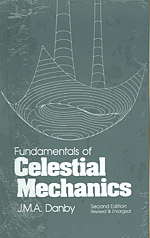Fundamentals of Celestial Mechanics, 2nd Ed. [J.M.A. Danby, 1992] (hardcover) 978-0943396200 J.M.A. Danby
List Price: \$29.95
Our Price: \$25.00
You Save: \$4.95 (17 %)

Detailed Description

4483 pgs, 1992, Willman-Bell Inc
ISBN 978-0943396200

This is an introductory text that should be accessible to readers having a background in calculus and elementary differential equations. The original edition (1962) has been radically revised, and emphasis is placed on computation. The numerical analysis needed for the computations is derived, and sample programs (run on a PC) are included.

There are introductory chapters on the astronomical background and on vectorial mechanics. Sections dealing with the problem of two bodies include the use of universal variables, several methods (including that of Laguerre) for solving Kepler's equation, and problems. The chapter on the determination of orbits includes two versions of Gauss' method, the application of least squares and an introduction to recursive methods. The chapter on numerical methods includes three methods for the numerical integration of differential equations, one of which has full step size control. There are also chapters on perturbations. the three- and n-body problems, the motion of the Moon and the rotations of the Earth and Moon.

The appendix includes numerical tables and derivations of properties of conic sections that are used in the text. The text includes several hundred problems and suggested computer projects. A diskette that can be used in conjunction with this text is separately available as detailed on the last page of this book.

1. The Astronomical Background
2. Introduction to Vectors
3. Introduction to Vectorial Mechanics
4. Central Orbits
5. Some Properties of Solid Bodies
6. The Two-Body System
7. The Determination of Orbits
8. The Three-Body Problem
9. The n-Body Problem
10. Numerical Procedures
11. Perturbations
12. The Motion of the Moon
13. The Earth and its Rotation
14. The Moon and its Rotation
15. Appendices
A: Properties of Conics
B: The Rotation of Axes
C: Numerical Values
D: Miscellaneous Expansions in Series
E: The Solution of Linear Systems
F: The Generation on the Computer of Gaussian Deviates
G: Some Orbits of Comets and Minor Planets
H: The Greek Alphabet
I: Random Variables, and Least Squares
J: Notes on Hamiltonian Mechanics he Astronomical Background